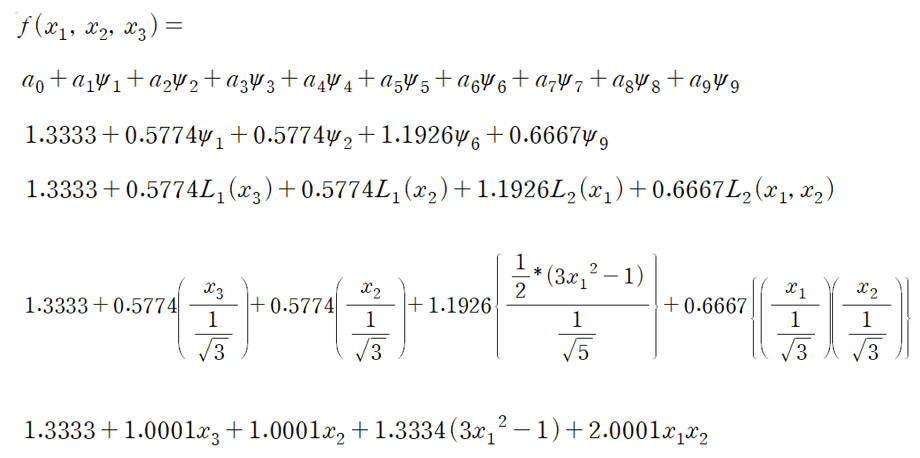# Definition of orthogonal polynomials

Hi UQLabI extract my PCE function after training using just data.
My input is consist of three variables and all distributions of variables are uniform distribution.

So. below expression is my result(PCE function)I confirmed that the correct result is obtained only when iusing ExpDesign.U (Orthogonal polynomial domain) not ExpDesign.X.
The research I am going to do is try to optimize using the PCE function, but when I do that, I get the U value, not the X values ​​at the optimal point.

1. Is there a way to find the X value by knowing the U value?
2. I have confirmed that there are different support ranges for uniform basis and other basis, but I don’t know the principle of changing X to U and U to X.
• My input variable is composed of -3 to 1, so it is outside the support range of the uniform distribution.
1 Like

Hi @Chemicaleng!

UQlab uses orthonormal polynomials that correspond to standard distributions - e.g., Legendre polynomials always correspond to X~U([-1,1]), and Hermite polynomials correspond to X~N(0,1).

If your input distribution is uniform, but not standard uniform, UQLab automatically takes care of the isoprobabilistic transform to the standard space. In your case, it’s a simple linear transformation from [-3,1] to [-1,1]. The values in U are the points of your experimental design transformed to standard space. The PCE that is built lives in standard space, as you observed correctly.

If you want to transform between your input space and the standard space, you can use the function `uq_GeneralIsopTransform` (see Input Manual, section 2.5). To get the input object of the standard space, you can either create it manually or get it from your PCE object: it is in `myPCE.ExpDesign.ED_Input` (see PCE Manual, table 16).

I hope this helps, good luck!